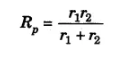# To Verify The Laws Of Combination (Parallel) Of Resistances Using A Metre Bridge

## Aim:

To verify the laws of combination (parallel) of resistances using a metre bridge

## Materials Required:

1. 2 different resistances (carbon or wire-wound resistors),
2. metre bridge,
3. galvanometer,
4. a cell or battery eliminator,
5. a jockey,
6. a rheostat,
7. a resistance box,
8. a plug key,
9. sandpaper and
10. thick connecting wires.

## Theory:

Consider two resistances, r1 and r2, are connected in series.

The series combination resistance RS is given by RS = r1 + r2

When connected in parallel, the resistance of the combination is given by Rp## Diagram

Resistance connected in ParallelResistances r1 and r2 connected in parallel to one arm of a metre bridge

## Procedure

1. Set up the circuit as shown in the figure above.
2. Connect R1 and R2 as shown in the figure.
3. Tighten all plugs in the resistance box by pressing and rotating each plug to assure that all plugs make good electrical connections. Using sandpaper, clean the ends of connecting wires before making the connections.
4. Remove some plug(s) from the resistance box to get the suitable value of resistance R
5. Get a null point D on the metre bridge wire by sliding the jockey between ends A and C.
6. Note the value of the resistance R and lengths AD and DC.
7. Calculate the experimental value of the equivalent parallel resistance
8. Repeat the experiment for four more values of resistance R. Obtain the mean value of unknown resistance.

## Observations And Calculations

 sl.no Resistance R (ohm) Resistance from the resistance box R (ohm) Length AD=l Length DC=100-1 Rp=100-1/l Mean resistance (ohm) R1 only 1 2 3 4 5 r1 = r2 only 1 2 3 4 5 r2 = r1 and r2 in parallel 1 2 3 4 5 Rp =

## Precautions

• Ensure the connection is neat, clean and tight
• Insert key only while taking an observation
• Move jockey gently over the metre bridge wire.

## Sources Of Error

• The instrument screw may be loose
• Unavailability of thick connecting wires

## Result

• The experimental value of Xp = ohm
• The theoretical value of Xp = ohm
• The experimental and theoretical value of Xp is found to be the same. Hence, the law of resistance in parallel is verified.

## Viva Questions

1. List the factors affecting the resistance?

ANS: The factors affecting the resistance are,

• Length
• material
• Area of cross-section
• The temperature of the conductors

2. What is the mathematical form of Ohm’s law?

ANS: V=IR

3. What is resistance?

ANS: The ratio of potential difference V across the ends of a conductor to the current I flowing through it. It is represented by R and is given by R=V/I

4. What is ohmic resistance?

ANS: The resistance which obeys ohms law is known as Ohmic resistance.

5. Give examples of non-ohmic resistance?

ANS: Transistors, vacuum tube diodes and semiconductor diodes.

Test Your Knowledge On To Verify The Laws Of Parallel Combination Of Resistances Using A Metre Bridge Experiment!